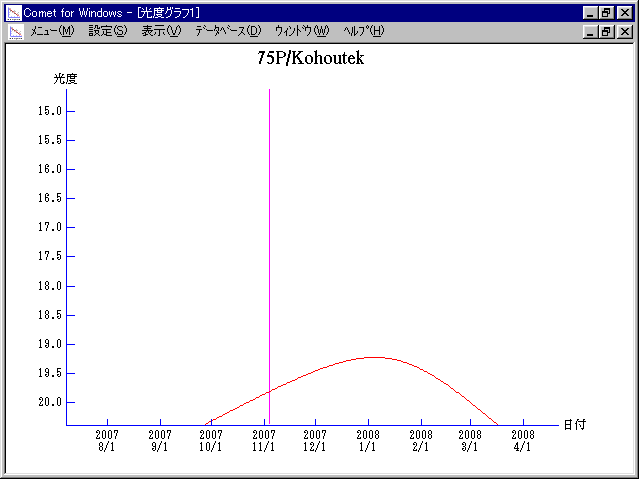# 75D/Kohoutek (2007)###Orbital Elements

```Perturbed ephemeris below is based on elements from MPC 31663.

Epoch = 2454400.50000 TT   T = 2454408.75183 TT
Peri. =  175.62701         a =     3.5528843 AU
Node  =  269.68312 J2000.0 e =     0.4946376
Incl. =    5.90138         q =     1.7954940 AU
```

###Magnitudes Graph

```        m1 = 18 + 5 log d + 5 log r
```##### The orbital elements are calculated using the Minor Planet & Comet Ephemeris Service. The magnitudes graphs are made with Comet for Windows.